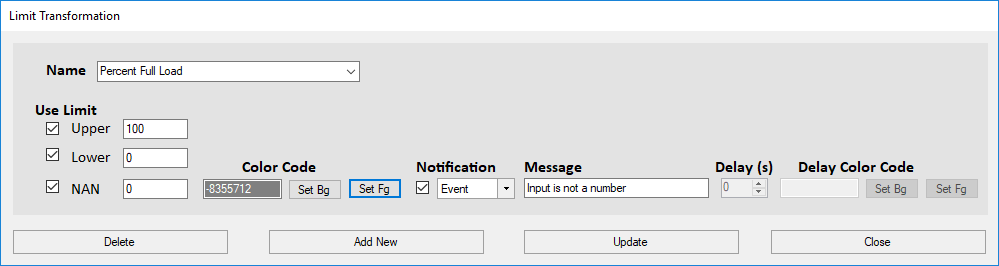The limit transformation converts an input based on upper and lower bounds. It also converts a non-number input into a number and can generate an alarm. Each limit is only applied if the checkbox next to it is checked.If the Input Value is greater than or equal to the upper bound and “Upper” is checked, the Output is set equal to the upper bound. If the Input Value is less than or equal to the lower bound and “Lower” is checked, the Output is set equal to the lower bound. If the input is in between the upper and lower bounds, the value remains unchanged. If “NAN” is checked, it converts any non-number into the number or text set for NAN. An alarm can be generated if the input is a non-number.